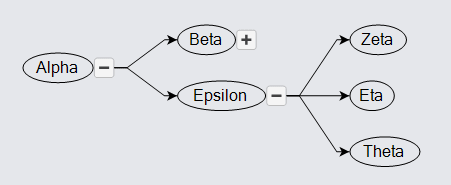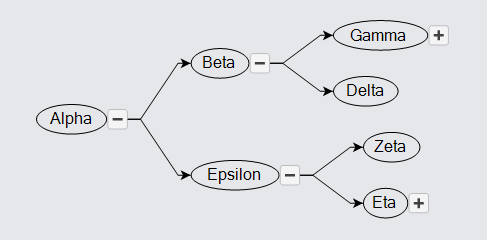# SubTrees

Tree diagrams can get very large. One way to simplify the diagram is to hide branches of the tree. "Collapsing" a tree node means making all of its children and the links to them not visible, and recursively collapsing all of the children that have children.

To collapse a node in a tree, set Node.IsTreeExpanded to false; to make sure it is expanded, set that property to true. You should not set this property to true on a Node that is not GraphObject.Visible.

It is commonplace to provide a button on a node to allow users to collapse and expand subtrees as they wish. GoDiagram makes this easy to implement by providing a predefined kind of Panel, named "TreeExpanderButton", that acts as a button to collapse and expand the subtree of a node. This button changes the visibility of all parts of the subtree except for the node itself.

Clicking on an expander button also invalidates the layout that is reponsible for the node. Collapsing a subtree often results in a large empty area, and expanding a subtree often results in overlapping nodes, so a new layout ought to be performed again to make the tree look better.

``````
diagram.NodeTemplate =
new Node("Horizontal")
new Panel("Auto")
new Shape("Ellipse") { Fill = null },
new TextBlock().Bind("Text", "Key")
),
Builder.Make<Panel>("TreeExpanderButton")
);

diagram.Layout = new TreeLayout();

diagram.Model =
new MyModel {
NodeDataSource = new List<NodeData> {
new NodeData { Key = "Alpha" }, new NodeData { Key = "Beta" },
new NodeData { Key = "Gamma", }, new NodeData { Key = "Delta" },
new NodeData { Key = "Epsilon" }, new NodeData { Key = "Zeta" },
new NodeData { Key = "Eta" }, new NodeData { Key = "Theta" }
},
new LinkData { From = "Alpha", To = "Beta" },
new LinkData { From = "Beta", To = "Gamma" },
new LinkData { From = "Beta", To = "Delta" },
new LinkData { From = "Alpha", To = "Epsilon" },
new LinkData { From = "Epsilon", To = "Zeta" },
new LinkData { From = "Epsilon", To = "Eta" },
new LinkData { From = "Epsilon", To = "Theta" }
}
};
``````If you were to first collapse the "Beta" node and then collapse the "Alpha" root node, if you then expanded the "Alpha" node, the "Beta" node would remain collapsed, whereas the "Epsilon" node would remain expanded. This is because the collapsing operation remembers the state of nodes within the subtree, as the property Node.WasTreeExpanded. The expanding operation respects the value of that property when recursing through the subtree.

You may also want to start off the tree mostly or completely collapsed. First, set Node.IsTreeExpanded to false in the template. That will cause only the roots of the tree to be shown. Second, if you want to show three levels of the tree, call Node.ExpandTree.

``````
diagram.NodeTemplate =
new Node("Horizontal") { IsTreeExpanded = false }  // default collapsed
new Panel("Auto")
new Shape("Ellipse") { Fill = null },
new TextBlock().Bind("Text", "Key")
),
Builder.Make<Panel>("TreeExpanderButton")
);

diagram.Layout = new TreeLayout();

diagram.InitialLayoutCompleted += (s, e) => {
var rit = e.Diagram.FindTreeRoots();
while (rit.MoveNext()) {
var r = rit.Current;
r.ExpandTree(3);
}
};

diagram.Model =
new MyModel {
NodeDataSource = new List<NodeData> {
new NodeData { Key = "Alpha" }, new NodeData { Key = "Beta" },
new NodeData { Key = "Gamma", }, new NodeData { Key = "Delta" },
new NodeData { Key = "Epsilon" }, new NodeData { Key = "Zeta" },
new NodeData { Key = "Eta" }, new NodeData { Key = "Theta" },
new NodeData { Key = "Iota" }, new NodeData { Key = "Kappa" },
new NodeData { Key = "Lambda" }
},
new LinkData { From = "Alpha", To = "Beta" },
new LinkData { From = "Beta", To = "Gamma" },
new LinkData { From = "Beta", To = "Delta" },
new LinkData { From = "Alpha", To = "Epsilon" },
new LinkData { From = "Epsilon", To = "Zeta" },
new LinkData { From = "Epsilon", To = "Eta" },
new LinkData { From = "Eta", To = "Theta" },
new LinkData { From = "Gamma", To = "Iota" },
new LinkData { From = "Iota", To = "Kappa" },
new LinkData { From = "Iota", To = "Lambda" }
}
};
``````